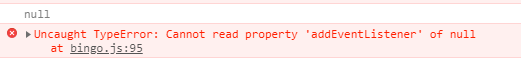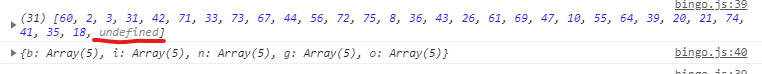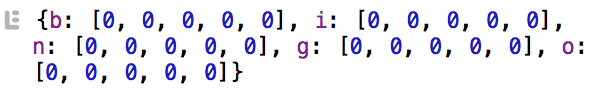Mikke.Learns

# Coding Project: Bingo. Part 4

May 4th, 2019

In order for us to check if the drawing function and player board function work, we need a button to press. So I made the projects first DOM-element.

`<button type="button" id="drawbutton">Draw a number</button>`

I then had to target the button in the JavaScript file. But somehow I could not get it to work.

`const drawButton = document.getElementById("#drawbutton");`After some trial and error, there was the familiar facepalm. We should of course not use a hashtag in the getElementById method.

`const drawButton = document.getElementById("drawbutton");`

So after fixing that, I was able to target the button.

I then attached the drawNumber function to the button by passing it in an event listener.

`drawButton.addEventListener("click", drawNumber);`

The drawing seemed to work. But I got undefined in the controllBoard array.I’m guessing it has something to do with the randomNumber variable inside our drawNumber function.

`let randomNumber = Math.floor((Math.random() * numbersToDraw.length) +1);`

Because when there are no numbers left in the numbersToDraw array, I also get undefined. So I bet the randomNumber sometimes pick a number outside the range of the numbersToDraw array.

This is the whole function:

```// Draw a random number from numbersToDraw const drawNumber = function () { // Pick a random number from numbersToDraw let randomNumber = Math.floor((Math.random() * numbersToDraw.length)); const entriesInPlayerBoard = Object.values(playerBoard); // Check player board for number for (var i = 0; i < entriesInPlayerBoard.length; i++) { if (entriesInPlayerBoard[i].indexOf(numbersToDraw[randomNumber]) != -1) { console.log('Match ' + entriesInPlayerBoard[i][entriesInPlayerBoard[i].indexOf(numbersToDraw[randomNumber])]); entriesInPlayerBoard[i][entriesInPlayerBoard[i].indexOf(numbersToDraw[randomNumber])] = 0; } } // We need to get the first item in the array we splice from numbersToDraw controllBoard.push(numbersToDraw.splice(randomNumber, 1)); }```

So how does this randomNumber function work? Math.random generates a floating-point random number between 0 and 1 (inclusive of 0, but not 1). An example would be 0.34635453463.

That’s not a number we want to work with. So we often use Math.random in conjunction with Math.floor and Math.ceil. to round the number.

Math.floor returns the largest integer less than or equal to a given number. Math.ceil does the opposite. It returns the smallest integer less than or equal to a number.

If we only use Math.floor(Math.random()), all we get is zero.

```// This returns 0 / zero console.log(Math.floor(Math.random()));```

But I have multiplied the random number with the length of the numbersToDraw array. So if the length is 75, we would get the following result using the example number above.

0.34635453463 * 75 = 5,19531801945

And if we wrap this in Math.floor() we would get 5. This tells us that we should get the number in the sixth place in the numbersToDraw array (arrays are zero-based).

Let’s use this and test the ends of our drawing function.

If there is one number left in the numbersToDraw (length 1), we would get this:

0.34635453463 * 1 = 0,34635453463

If we wrap that in Math.floor, we would get zero, and we would then get our last number in the target array: numbersToDraw.

If the target array is full (length 75) and the random number is the highest, we would get this:

0,99999999999 * 75 = 74,99999999925‬

If we wrap that in Math.floor, we would get 74, which would be the last number in the target numbersToDraw array.

This seems to be correct. But in our function, I’ve (for some reason, most likely copy/paste) added a 1.

`let randomNumber = Math.floor((Math.random() * numbersToDraw.length) +1);`

That would make the examples above 1 and 75 instead of 0 and 74. That’s what’s causing the undefined.

I removed it and voila! It works!

And as a bonus I see that the player board is completely cleared when all the numbers are drawn. Awesome!Our complete code so far:

```// The amount of number pieces let numberPieces = 75; // The numbers to draw from let numbersToDraw = [] // The control board let controllBoard = [] // The draw button const drawButton = document.getElementById("drawbutton"); // Fill the numbersToDraw array with numbers while (numberPieces > 0) { numbersToDraw.unshift(numberPieces); numberPieces--; } // Draw a random number from numbersToDraw const drawNumber = function () { // Pick a random number from numbersToDraw let randomNumber = Math.floor((Math.random() * numbersToDraw.length)); const entriesInPlayerBoard = Object.values(playerBoard); // Check player board for number for (var i = 0; i < entriesInPlayerBoard.length; i++) { if (entriesInPlayerBoard[i].indexOf(numbersToDraw[randomNumber]) != -1) { console.log('Match ' + entriesInPlayerBoard[i][entriesInPlayerBoard[i].indexOf(numbersToDraw[randomNumber])]); entriesInPlayerBoard[i][entriesInPlayerBoard[i].indexOf(numbersToDraw[randomNumber])] = 0; } } // We need to get the first item in the array we splice from numbersToDraw controllBoard.push(numbersToDraw.splice(randomNumber, 1)); console.log(controllBoard); console.log(playerBoard); //console.log(Math.floor(Math.random() * 75)); console.log(randomNumber); } // The player board let playerBoard = { b: [], i: [], n: [], g: [], o: [] } // Create a random number between X - X+15 to use in filling the player board const randomNumber = function (startNumber) { return Math.floor((Math.random() * 15) + startNumber); } // A function to fill a row in the player board const fillPlayerBoard = function (rowInPlayerBoard, number) { // Create a random number randomNum = randomNumber(number); counter = 0; // While loop to make sure that there is no duplicate numbers while (counter < 5) { if (rowInPlayerBoard.indexOf(randomNum) == -1) { rowInPlayerBoard.push(randomNum); counter++ } else { randomNum = randomNumber(number); } } // Make the middle of the player board "free" if (rowInPlayerBoard == playerBoard.n) { playerBoard.n = 0; } } fillPlayerBoard(playerBoard.b, 1); fillPlayerBoard(playerBoard.i, 16); fillPlayerBoard(playerBoard.n, 31); fillPlayerBoard(playerBoard.g, 46); fillPlayerBoard(playerBoard.o, 61); // Connecting the draw button to the draw function drawButton.addEventListener("click", drawNumber);```